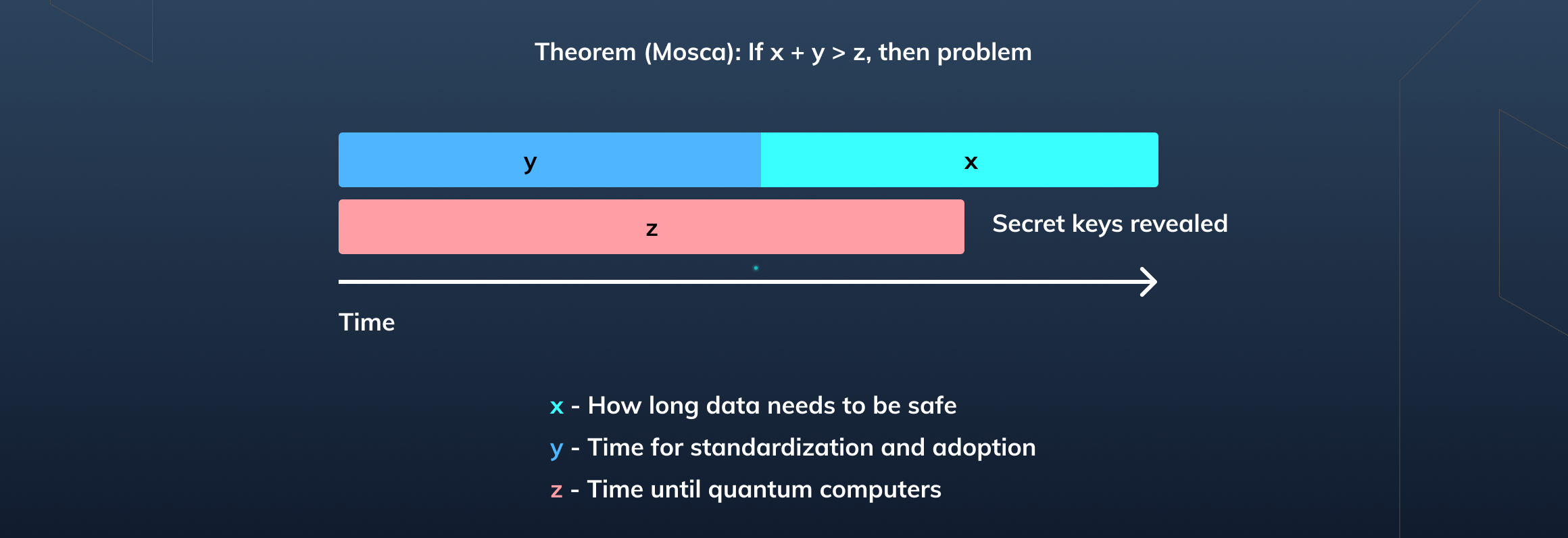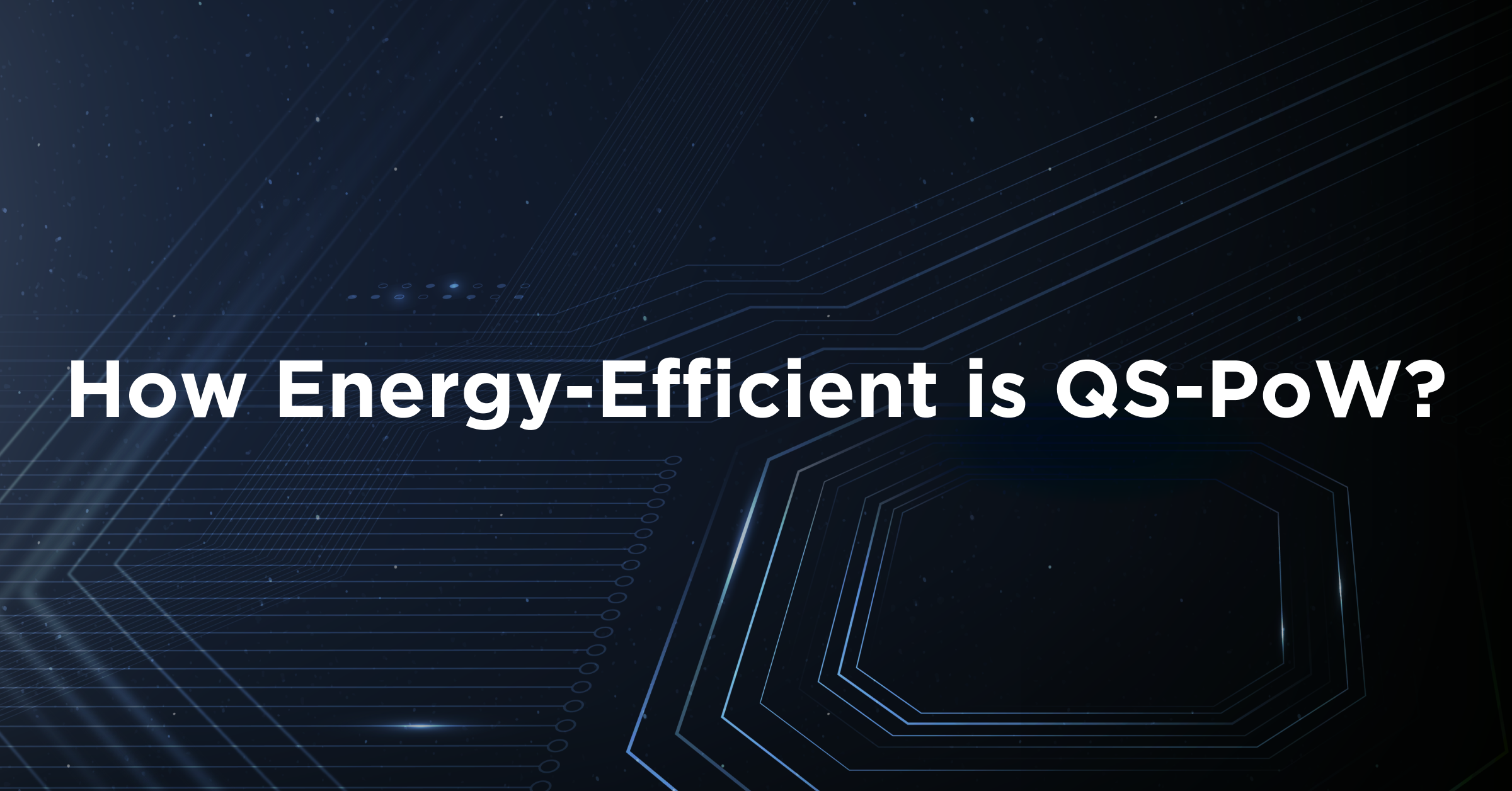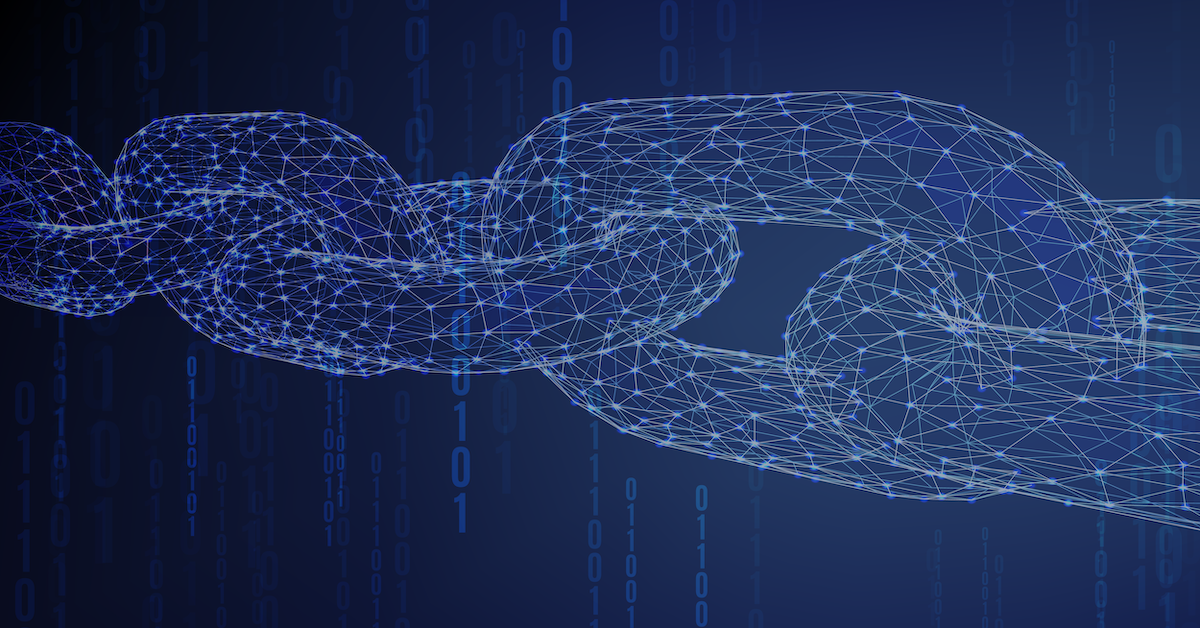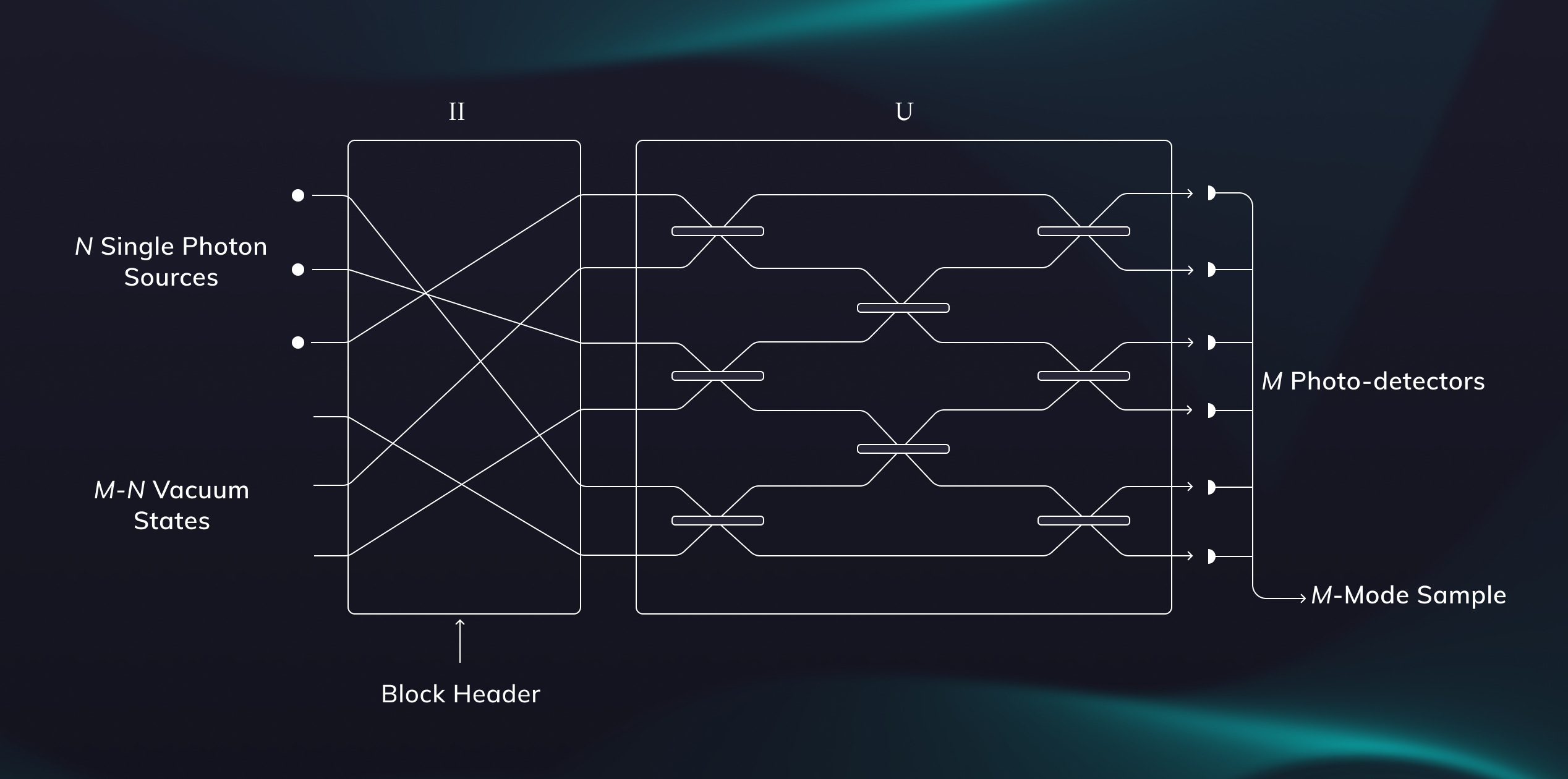### Growing threat of quantum computers

In recent years, substantial progress has been made in the field of quantum computing - a computing paradigm which leverages quantum mechanics to solve mathematical problems that are difficult and often intractable on conventional machines. Quantum computers are capable of solving certain problems classical computers are incapable of solving in any feasible amount of time by encoding information into quantum bits (qubits). Each qubit doubles the amount of memory space available to execute algorithms, leading to vast improvements in computational time and performance. In November 2021 IBM unveiled a 127-qubit quantum processor, improving over its 65-qubit processor from the previous year . IBM plans to scale its quantum processors to over 1000 qubits by the end of 2023 with plans to eventually roll-out quantum processors wielding millions of qubits .

### Public-key cryptography is at risk

Public-key cryptography is particularly vulnerable to quantum computers. Two particularly prevalent families of public-key cryptography are elliptic-curve cryptography (ECC) and the RSA cryptosystem. ECCs security depends on the difficulty of computing discrete logarithms of groups of points over elliptic curves while the RSA cryptosystem relies on the difficulty of factoring large prime numbers. Both ECC and RSA are vulnerable to attacks via quantum computers. Shor’s algorithm is a quantum algorithm for efficiently finding the prime factors of sufficiently large integers - the very problem ECC and RSA rely on as being difficult to solve. This opens up attack vectors to a number of applications using public-key cryptography, such as Transport Layer Security (TLS), S/MIME, PGP, GPG, and digital signatures. If Shor’s algorithm were executed on a quantum computer with a sufficient number of qubits it would be capable of breaking the Elliptic Curve Digital Signature Algorithm (ECDSA), the public-key signature algorithm underlying Bitcoin, Ethereum, and many other blockchains.

Experts estimates of likelihood of a quantum computer breaking RSA-2048

### NIST Post-Quantum Cryptography competition

Post-quantum cryptography (PQC) is an active area of research that advances the use of quantum-resistant primitives in cryptographic algorithms. It's goal is to secure our digital infrastructure against both classical and quantum algorithms. An important consideration for PQC is interoperability with existing technologies so that digital infrastructure can be updated for the next wave of cryptographic standards. Post-quantum cryptography is a vital part of the response to the imminent threat of quantum computers such that in 2016 the National Institute of Standards and Technology (NIST) initiated a process to standardize a set of quantum-resistant public-key cryptographic algorithms, recognizing that current NIST approved algorithms are vulnerable to attacks from large-scale quantum computers. The first round saw a wide range of applicants grouped into five main categories:

1. Multivariate cryptography
2. Hash-based cryptography
3. Code-based cryptography
4. Isogeny-based cryptography
5. Lattice-based cryptography

Now in the third round, the digital signature algorithm field has been narrowed down to three finalists.

• CRYSTALS-DILITHIUM - A lattice based algorithm that is strongly secure based on the hardness of lattice problems over module lattices.
• FALCON - A lattice based algorithm based on the hard problem of short integer solutions over NTRU lattices, resulting in short signatures and fast implementations.
• RAINBOW - A multivariate digital signature algorithm with layered Unbalanced Oil and Vinegar (UOV) structures.

NIST PQC 3rd round finalists

### Rainbow has been broken

Rainbow was an attempt to improve the famous Unbalanced Oil and Vinegar (UOV) approach of making a multivariate trapdoor known for generating impractically large public keys. The public key of a UOV structure is a set of quadratic equations over two kinds of hidden variables: oil and vinegar variables. Since solving such equations appears to be hard even on large quantum computers, a trapdoor is required in order to generate valid signatures. In UOV and its derivatives, the signer assigns some random values to the vinegar variables and obtains the solutions to the oil variables by solving some linear equations. Then the best known attacks are analyzed, leading to the minimum number of equations and variables in order to have a secure signature scheme. Still, UOV has not achieved widespread use because of its very long key lengths. UOV public keys involve entire systems of equations spanning several hundred kilobytes of memory. Storing and communicating these large public keys can be extremely expensive on memory and communication resources at scale rendering it impractical for many applications. UOV was a good starting point but needed to have its key sizes reduced. Rainbow generalized UOV by introducing more layers of oil and vinegar structures. This enabled Rainbow to use a smaller set of equations, thereby reducing the number of variables in the system and reducing the public key size. However, this also opened up the digital signature algorithm scheme to new attack vectors. For example, the extra layers of UOV structures allow an attacker to guess on a smaller set of hidden variables and solve a much smaller set of equations than they would when attacking UOV. Rainbow could of course improve its security by increasing the size of the parameters, but then public key sizes and signing times would be slower than that of traditional UOV structures, in which case it would be better to use a simpler scheme instead of a more complicated scheme that is more expensive and less well-understood. The attack described above was executed in practice with private-keys being recovered on an average of 53-hours of computation on a standard laptop , proving the proposed parameter set for Rainbow is not secure.## Latest Research & Insights

See whats new in the quantum technologyOctober 31, 2023
How Energy-Efficient is QS-PoW?
Unlike Classical Blockchains like Bitcoin, which use massive amounts of energy (gas fees) to solve one-way hash functions on each node, BTQ’s QPOW is energy-efficient and post-quantum secure. The QPOW protocol’s energy consumption is determined by the number of samples per each Boson Sampler in the network. In this article, we make an estimate of energy consumption by the network in comparison to today’s Bitcoin’s energy-intensive protocol.